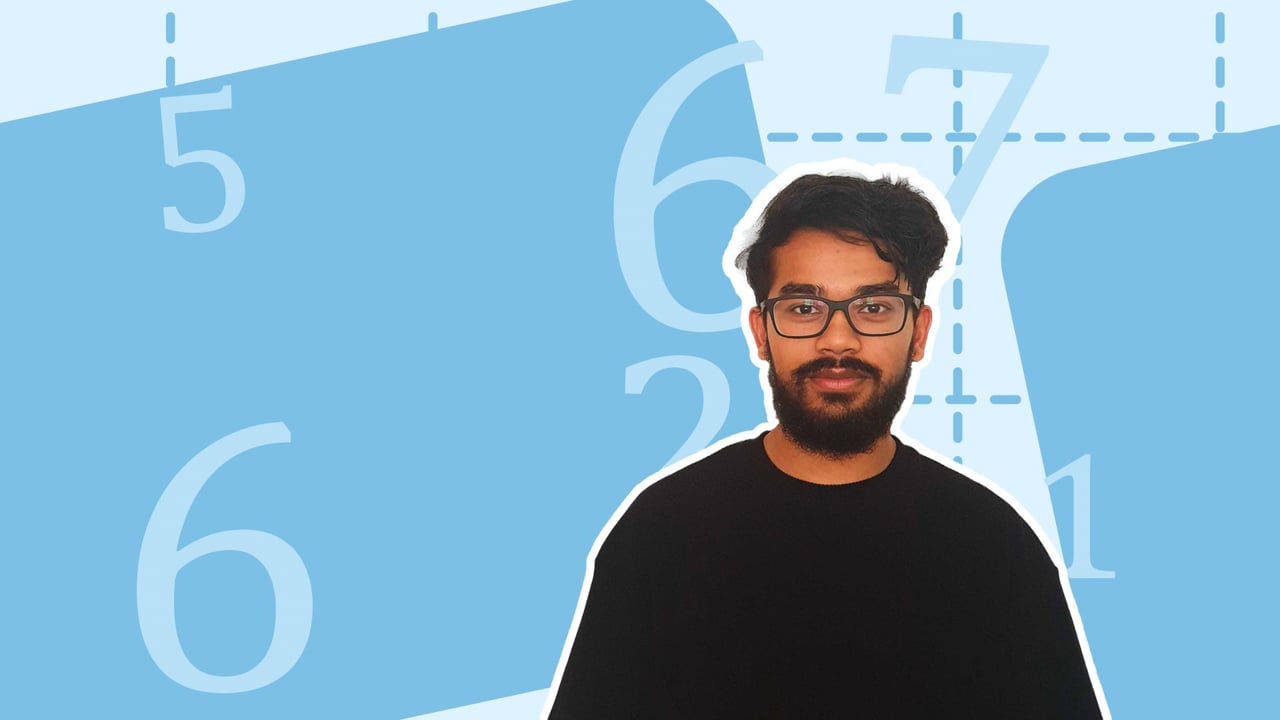Chapter OverviewMaths

Number and place value

Multiplication and division

Fractions

Measurement

Geometry - properties of shapes

Statistics

Maths

# Correspondence problems0%

Summary

# Correspondence problems

## ​​In a nutshell

Correspondence involves two or more connected items. In mathematics, correspondence problems are used to find the total number of combinations which can be made between connected things.

## Listing combinations

Tables are used to  identify all the possible combinations which can be made between groups of items, in order to solve correspondence problems.

##### Example 1

Vanessa has $3$ fruits and $2$ desserts. She would like to have $1$ of each. What combinations of fruit and dessert can Vanessa have?

 Fruit Dessert Grapes Chocolate Apple Chocolate Tomato Chocolate Grapes Doughnut Apple Doughnut Tomato Doughnut

Count the total number of combinations or rows in the table.

There are six different combinations of fruit and dessert Vanessa can make.

Note: Find all the combinations that can be made with one item first, then move onto the next item.

## Correspondence problems using multiplication

Sometimes, in correspondence problems, there are too many combinations to list in a table. Instead, multiplication can be used to find the total number of combinations.

##### Example 2

Ronaldo wants a drink and a snack. He can choose from $5$ drinks and $3$​ snacks. How many combinations of drinks and snacks can be made?

There are $5$​ drinks.

Each drink can be paired with $3$different snacks.

This means, there are $5$ lots of $3$ combinations between drinks and snacks.

$\underline{5 \times 3 = 15}$ combinations

FAQs

• Question: What method can be used to quickly solve correspondence problems?

Answer: Multiplication can be used to find the total number of combinations.

• Question: Why are tables used in correspondence problems?

Answer: Tables can be used to identify all the possible combinations which can be made between groups of items.

• Question: Why are correspondence problems used?

Answer: Correspondence problems are used to find the total number of combinations.

Theory

Exercises